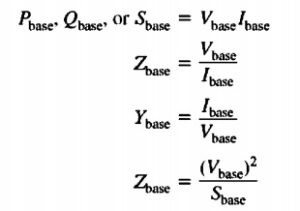Hi readers welcome to the new post. In this post, we will have a detailed look at Per-Unit System of Measurements. In the case of power system analysis used in electric, the per-unit system is the parameters of different terms that used as the base of the system. Due to this system, the measurements become easy. In such system where numerous transformer has employed the calculations of quantities become easy.

The basic configuration of a per-unit system is to get large differences in different parameters to get basic relations. So denotations of components in the network having per unit parameters are easy to handle. This system offered the unit denotation for voltage, current, power, and other electrical terms. Here we cover its different parameters related to the per-unit system. So let get started with Per-Unit System of Measurements.

## Per-Unit System of Measurements

• There are such circuits that comprise transformer are difficult to solve since there is a need to refer to voltage values used in the circuitry on sides of transformers.
• After referring the circuit is solved for measurements of current and voltage values.
• Here is a different system that used to the circuitry solution comprised of a transformer that deleted the requirement for voltage values transformation at each transformer in the circuitry.
• In place of transformation are resolved through techniques through needing the impedance conversion. Since every impedance conversion can be diminished circuitry comprises numerous conversion can be resolved to have minor faults possibility.
• This way of measurement is called a per-unit system.
• Here is another benefit of the per-unit system is efficient for electrical machines and transformers. Since the physical dimensions of these machines change it inner impedance changes.
• So primary circuitry reactance of 0.1 ohms can be large in the case of a single transformer and less for others. It relies at the voltage and power values of devices.
• Though it turning out that in the case of the per-unit system corresponding to the rating of transformer and impedances relies in close values of every category and structure of the machine.
• In per unit system, the voltage-current power and impedances and other electric parameters are not found in normal system international.
• In place, every electric parameter is calculated in a decimal fraction of the base level.
• The parameters can be defined at the per unit through this expression.

Quantity per unit=Actual value/base value of quantity• Here actual value is a value given in vols ampere and ohms,
• It easy to select 2 base quantities to explain the per-unit system. The term normally choose are voltage and power,
• When these parameters are chosen other base parameters according to these terms are according to normal electric terms.
• In one phase system, these expressions are mentioned here.• After base values of S P and V selection, other base parameters are measured with the use of the above-given equation.
• In a power system, the base apparent power and voltage are chosen at a certain location of the system.
• The transform has no fact at the base apparent power. because of apparent power in a transformer like or equal to the apparent power of transformer.
• At other terms, the voltage varies when moving to the transformer. Therefore parameters Vbase varies at each transformer in the network following the turns ratio.
• Since the base terms vary in the transformer the procedure of referred terms to the communal voltage parameters is considered in the per unit transformation